User’s Guide : Multiple Equation Analysis : Models : Working with the Model Structure

Working with the Model Structure
As with other objects in EViews, we can look at the information contained in the model object in several ways. Since a model is a set of equations that describe the relationship between a set of variables, the two primary views of a model are the equation view and the variable view. EViews also provides two additional views of the structure of the model: the block view and the text view.
Equation View
The equation view is used for displaying, selecting, and modifying the equations contained in the model. An example of the equation view can be seen on (here).
Each line of the window is used to represent either a linked object or an inline text equation. Linked objects will appear similarly to how they do in the workfile, with an icon representing their type, followed by the name of the object. Even if the linked object contains many equations, it will use only one line in the view. Inline equations will appear with a “TXT” icon, followed by the beginning of the equation text in quotation marks.
The remainder of the line contains the equation number, followed by a symbolic representation of the equation, indicating which variables appear in the equation.
Any errors in the model will appear as red lines containing an error message describing the cause of the problem.
You can open any linked objects directly from the equation view. Simply select the line representing the object using the mouse, then choose Open Link from the right mouse button menu.
The contents of a line can be examined in more detail using the equation properties dialog. Simply select the line with the mouse, then choose Properties… from the right mouse button menu. Alternatively, simply double click on the object to call up the dialog.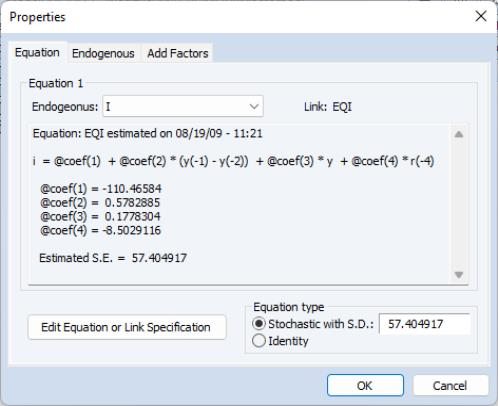For a link to a single equation, the dialog shows the functional form of the equation, the values of any estimated coefficients, and the standard error of the equation residual from the estimation. If the link is to an object containing many equations, you can move between the different equations imported from the object using the Endogenous list box at the top of the dialog. For an inline equation, the dialog simply shows the text of the equation.
The Edit Equation or Link Specification button allows you to edit the text of an inline equation or to modify a link to point to an object with a different name. A link is represented in text form as a colon followed by the name of the object. Note that you cannot modify the specification of a linked object from within the model object, you must work directly with the linked object itself.
In the bottom right of the dialog, there are a set of fields that allow you to set the stochastic properties of the residual of the equation. If you are only performing deterministic simulations, then these settings will not affect your results in any way. If you are performing stochastic simulations, then these settings are used in conjunction with the solution options to determine the size of the random innovations applied to this equation.
The Stochastic with S.D. option for Equation type lets you set a standard deviation for any random innovations applied to the equation. If the standard deviation field is blank or is set to “NA”, then the standard deviation will be estimated from the historical data. The Identity option specifies that the selected equation is an identity, and should hold without error even in a stochastic simulation. See “Stochastic Options” below for further details.
The equation properties dialog also gives you access to the property dialogs for the endogenous variable and add factor associated with the equation. Simply click on the appropriate tab. These will be discussed in greater detail below.
In addition, the equation view offers search capability, allowing you to quickly locate and work with an equation of interest. You may access equation find access it either by clicking the Find button (on the far right of the button bar), or pressing CTRL-F to bring up the Model Find dialog: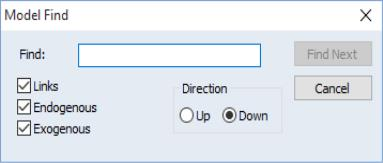You may search for equations by name, by exogenous variable or by endogenous variable. For example if you have a linked equation called EQ01, you can type “Eq01” in the Find edit field, click on Find Next, and EViews will select that equation in the equation view. Similarly you can type in GDP, and EViews will select the next equation (either linked or written as an inline expression in the model) that contains the variable GDP.
The check boxes on the left allow you to modify which type of objects/variables to search. If we unselect Exogenous, and then search for GDP, EViews will find only equations which contain GDP as an endogenous variable.
Variable View
The variable view is used for adjusting options related to variables and for displaying and editing the series associated with the model (see the discussion in “Examining the Solution Results”). The variable view lists all the variables contained in the model, with each line representing one variable. Each line begins with an icon classifying the variable as endogenous, exogenous or an add factor. This is followed by the name of the variable, the equation number associated with the variable, and the description of the variable. The description is read from the associated series in the workfile.
Note that the names and types of the variables in the model are determined fully by the equations of the model. The only way to add a variable or to change the type of a variable in the model is to modify the model equations.
You can adjust what is displayed in the variable view in a number of ways. By clicking on the Filter/Sort button just above the variable list, you can choose to display only variables that match a certain name pattern, or to display the variables in a particular order. For example, sorting by type of variable makes the division into endogenous and exogenous variables clearer, while sorting by override highlights which variables have been overridden in the currently active scenario.
The variable view also allows you to browse through the dependencies between variables in the model by clicking on the Dependencies button. Each equation in the model can be thought of as a set of links that connect other variables in the model to the endogenous variable of the equation. Starting from any variable, we can travel up the links, showing all the endogenous variables that this variable directly feeds into, or we can travel down the links, showing all the variables upon which this variable directly depends. This may sometimes be useful when trying to find the cause of unexpected behavior. Note, however, that in a simultaneous model, every endogenous variable is indirectly connected to every other variable in the same block, so that it may be hard to understand the model as a whole by looking at any particular part.
You can quickly view or edit one or more of the series associated with a variable by double clicking on the variable. For several variables, simply select each of them with the mouse then double click inside the selected area.
Block Structure View
The block structure view of the model analyzes and displays any block structure in the dependencies of the model.
Block structure refers to whether the model can be split into a number of smaller parts, each of which can be solved for in sequence. For example, consider the system:

 block 1 x = y + 4 y = 2*x – 3 block 2 z = x + y
Because the variable Z does not appear in either of the first two equations, we can split this equation system into two blocks: a block containing the first two equations, and a block containing the third equation. We can use the first block to solve for the variables X and Y, then use the second block to solve for the variable Z. By using the block structure of the system, we can reduce the number of variables we must solve for at any one time. This typically improves performance when calculating solutions.
Blocks can be classified further into recursive and simultaneous blocks. A recursive block is one which can be written so that each equation contains only variables whose values have already been determined. A recursive block can be solved by a single evaluation of all the equations in the block. A simultaneous block cannot be written in a way that removes feedback between the variables, so it must be solved as a simultaneous system. In our example above, the first block is simultaneous, since X and Y must be solved for jointly, while the second block is recursive, since Z depends only on X and Y, which have already been determined in solving the first block.
The block structure view displays the structure of the model, labeling each of the blocks as recursive or simultaneous. EViews uses this block structure whenever the model is solved. The block structure of a model may also be interesting in its own right, since reducing the system to a set of smaller blocks can make the dependencies in the system easier to understand.
Text View
The text view of a model allows you to see the entire structure of the model in a single screen of text. This provides a quick way to input small models, or a way to edit larger models using copy-and-paste.
The text view consists of a series of lines. In a simple model, each line simply contains the text of one of the inline equations of the model. More complicated models may contain one of more of the following:
A line beginning with a colon “:” represents a link to an external object. The colon must be followed by the name of an object in the workfile. Equations contained in the external object will be imported into the model whenever the model is opened, or when links are updated.
where endogenous_name is the name of the endogenous variable of the equation to which the add factor will be applied, and add_name is the name of the series. The option (v) is used to specify that the add factor should be applied to the endogenous variable. The default is to apply the add factor to the residual of the equation. See “Using Add Factors” for details.
A line beginning with “@INNOV”' specifies an innovation variance. The innovation variance has two forms. When applied to an endogenous variable it has the form:
@innov endogenous_name number
where endogenous name is the name of the endogenous variable and number is the standard deviation of the innovation to be applied during stochastic simulation. When applied to an exogenous variable, it has the form:
@innov exogenous_name number_or_series
where exogenous name is the name of the exogenous variable and number_or_series is either a number or the name of the series that contains the standard deviation to be applied to the variable during stochastic simulation. Note that when an equation in a model is linked to an external estimation object, the variance from the estimated equation will be brought into the model automatically and does not require an @innov specification unless you would like to modify its value.
The keyword “@TRACE”, followed by the names of the endogenous variables that you wish to trace, may be used to request model solution diagnostics. See “Diagnostics”.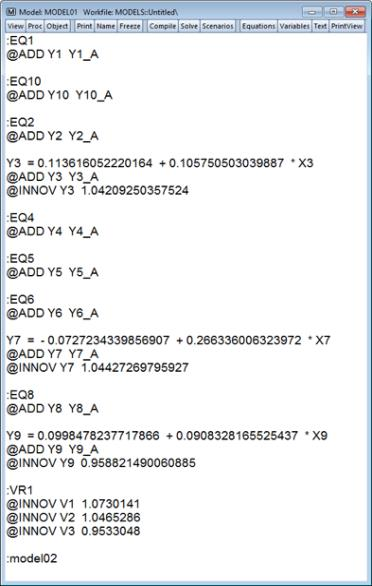Users of early versions of EViews should note that two commands that were previously available, @assign and @exclude, are no longer part of the text form of the model. These commands have been removed because they now address options that apply only to specific model scenarios rather than to the model as a whole. When loading in models created by earlier versions of EViews, these commands will be converted automatically into scenario options in the new model object.
Print View
Print view (available from the PrintView button, or from View/Print View) offers a new way of looking at the underlying equations in your model. While similar to text view, print view offers display enhancements, but does not allow editing of the model. Selecting print view will bring up the Model Print View dialog: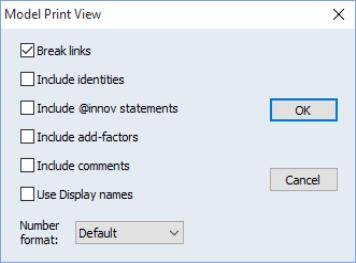The dialog settings let you determine how you would like to view the equations.
Checking the Break links check box tells EViews to display linked equations as expressions rather than as linked objects. Note that this is only for display—the linked equations will remain linked in your model, but will be displayed as though they were broken.
The Include identities, Include @innov statements, Include add-factors, and Include comments check boxes determine whether the respective model elements will be displayed in the view.
Checking the Use Display names check box will replace all series names in the model with their display name.
The Number format dropdown allows you to specify the display format for coefficients in the equations.
For example, the traditional text view of the model presented in “Text View” may be compared to the print view which substitutes embedded equation and model definitions and displays fewer significant digits: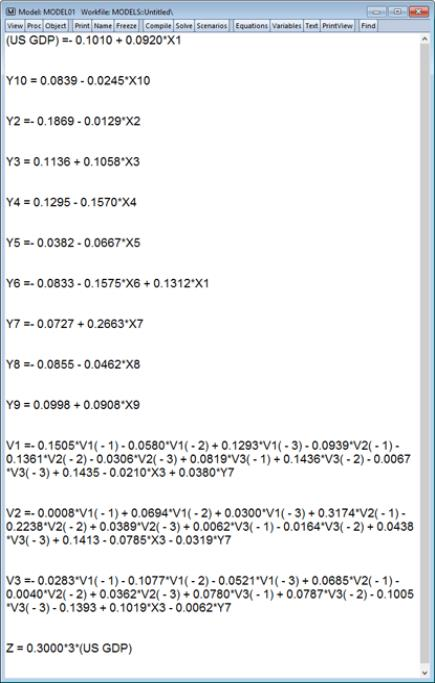Dependency Graph View
This view provides a graphical representation of the relationships among the model's variables. The model is shown as a directed graph with nodes for each variable and edges between pairs of related variables. Edges follow the direction of information flow. For example, in the below graph variable NOW depends on variable HILO (the equation for NOW includes HILO), resulting in a directed edge from HILO to NOW. Variables may be mutually dependent, in which case the edge between them will have arrowheads at both ends, e.g., variables BTE and XBTE.
Computing the layout for the graph is computationally expensive and may take some time. If you select this view but wish to abort before the layout calculations are completed, hit the F1 key.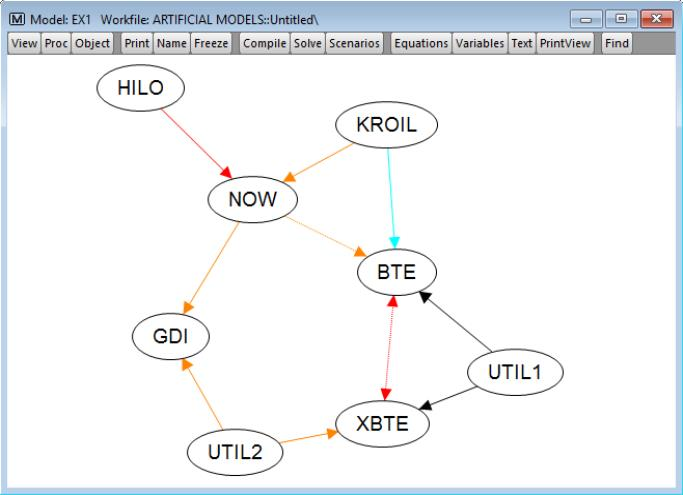The color of each edge summarizes the temporal (lag/lead) attributes of the dependency along a warm-cool spectrum:
Orange: Only lagged values are present.
Red: Lagged and contemporaneous values are present.
Black: Only contemporaneous values are present.
Blue: Lead and contemporaneous values are present.
Cyan: Only lead values are present.
If both lagged and lead values are present, the edge is shown in a either light or dark purple. If a lag or lead of length four or more is present, the edge is shown as a dotted line, e.g., the edge between variables BTE and XBTE.